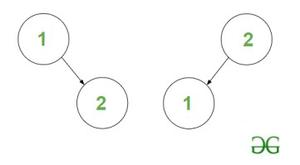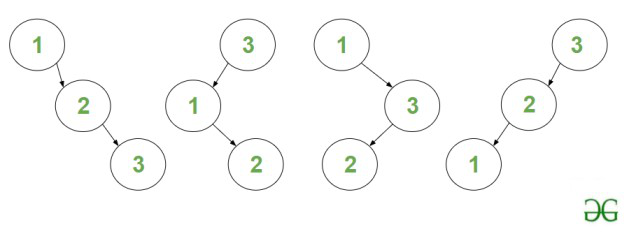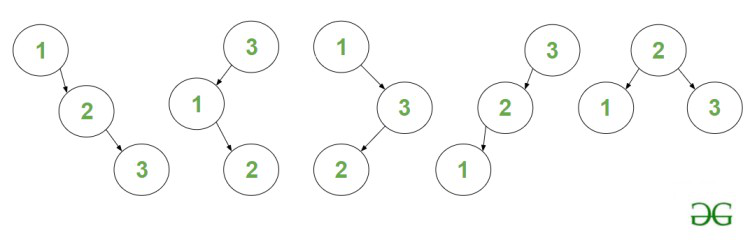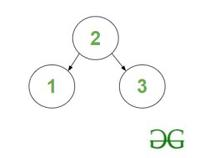# Count of BSTs having N nodes and maximum depth equal to H

Given two integers N and H, the task is to find the count of distinct Binary Search Trees consisting of N nodes where the maximum depth or height of the tree is equal to H

Note: The height of BST with only the root node is 0.

Examples:

Input: N = 2, H = 1
Output: 2
Explanation: The two BST’s are :BST’s of height H = 1 and nodes N = 2

Input: N = 3, H = 2
Output: 4
Explanation: The four BST are :BST’s of height H = 2 and nodes N = 3

Naive Approach: The problem can be solved using Recursion which can be memoized to obtain a Dynamic Programming solution based on the following idea:

The problem can be efficiently solved by finding the count of BST’s having maximum depth upto H (i.e., [0 – H]) instead of exactly H.

Let f(N, H) represent the count of BST’s consisting of ‘N’ nodes and having maximum depth upto ‘H’. Then the solution for the above problem: count of BST’s having maximum depth of exactly ‘H’ is equal to f(N, H) – f(N, H – 1)

Follow the illustration below for a better understanding.

Illustration:

Consider: N = 3, H = 2

The answer for this example is : count of BST’s of maximum depth upto 2 –  count of BST’s of maximum depth upto 1.

• Count of BST’s of maximum depth upto 2 is 5, they are:5 – BST’s of maximum depth upto 2

• Count of BST’s of maximum depth upto 1 is 1, it is :1 – BST of maximum depth upto 1

• Hence the count of BST’s of maximum depth equal to ‘2’ is 4.

Follow the steps mentioned below to solve the problem.

• The count of BST with Node i as root Node is equal to product of count of BST’s of left subtree formed by nodes 1 to i-1 and right subtree formed by nodes i+1 to N.
• In order to find the count of BST of left subtree, we can recursively call the same function for depth H-1 and  N=i – 1. To find the count of BST of right subtree, recursively call the function for depth H-1 and N=N-i.
• Loop over all values of i from [1, N] as root node and add the product of count of left and right subtree to the result.

Time Complexity: O(N * 2N)
Auxiliary Space: O(1)

Efficient Approach: The above approach can be optimized by using Dynamic Programming because the above problem has Overlapping subproblems and an Optimal substructure. The subproblems can be stored in dp[][] table memoization where dp[N][H] stores the count of BST of maximum depth up to H consisting of N nodes. Follow the steps below to solve the problem:

• Initialize a global multidimensional array dp with all values as -1 that stores the result of each recursive call.
• Define a recursive function, say countOfBST(N, H) and perform the following steps.
• Case 1: If N = 0, return 1.
• Case 2: If H = 0, return true if N = 1.
• If the result of the state dp[N][H] is already computed, return this value dp[N][H].
• Iterate over the range [1, N] using the variable ‘i‘ as root and perform the following operations.
• Multiply the value of recursive functions countOfBST(i – 1, H – 1) and countOfBST(N – i, H – 1). The two functions calculate the count of BST for the left and the right subtree respectively.
• Add the term to the final answer which stores the total count of BSTs possible for all roots from [1, N].
• Print the value returned by the function countOfBST(N, H).

Below is the implementation of the above approach :

## C++

 `// C++ code to implement the approach`   `#include ` `using` `namespace` `std;`   `// Declaring a dp-array` `int` `dp;`   `const` `int` `mod = 1000000007;`   `// Function to find the count of` `// BST upto height 'H' consisting` `// of 'N' nodes.` `int` `countOfBST(``int` `N, ``int` `H)` `{`   `    ``// Base Case1 : If N == 0, return` `    ``// 1 as a valid BST has been formed` `    ``if` `(N == 0) {` `        ``return` `1;` `    ``}`   `    ``// Base Case2 : If H == 0, return true` `    ``// if N == 1` `    ``if` `(H == 0) {` `        ``return` `N == 1;` `    ``}`   `    ``// If the current state has already` `    ``// been computed, then return it.` `    ``if` `(dp[N][H] != -1) {` `        ``return` `dp[N][H];` `    ``}`   `    ``// Initialize answer to 0.` `    ``int` `ans = 0;`   `    ``// Iterate over all numbers from` `    ``// [1, N], with 'i' as root.` `    ``for` `(``int` `i = 1; i <= N; ++i) {`   `        ``// Call the recursive functions to` `        ``// find count of BST of left and right` `        ``// subtrees. Add the product of` `        ``// both terms to the answer.` `        ``ans += (countOfBST(i - 1, H - 1) * 1LL` `                ``* countOfBST(N - i, H - 1))` `               ``% mod;`   `        ``// Take modulo 1000000007` `        ``ans %= mod;` `    ``}`   `    ``// Return ans` `    ``return` `dp[N][H] = ans;` `}`   `// Utility function to find the count` `// of BST upto height 'H' consisting` `// of 'N' nodes.` `int` `UtilCountOfBST(``int` `N, ``int` `H)` `{`   `    ``// Initialize dp-array with -1.` `    ``memset``(dp, -1, ``sizeof` `dp);`   `    ``// If height is 0, return true if` `    ``// only one node is present.` `    ``if` `(H == 0) {` `        ``return` `(N == 1);` `    ``}`   `    ``// Function call.` `    ``return` `(countOfBST(N, H)` `            ``- countOfBST(N, H - 1)` `            ``+ mod)` `           ``% mod;` `}`   `// Driver code` `int` `main()` `{` `    ``// Number of nodes` `    ``int` `N = 3;`   `    ``// Height of tree` `    ``int` `H = 2;`   `    ``cout << UtilCountOfBST(N, H) << endl;` `    ``return` `0;` `}`

## Java

 `// Java implementation of above approach` `import` `java.io.*;` `import` `java.util.*;`   `class` `GFG {`   `  ``// Declaring a dp-array` `  ``static` `int``[][] dp = ``new` `int``[``105``][``105``];`   `  ``static` `int` `mod = ``1000000007``;`   `  ``// Function to find the count of` `  ``// BST upto height 'H' consisting` `  ``// of 'N' nodes.` `  ``static` `int` `countOfBST(``int` `N, ``int` `H)` `  ``{`   `    ``// Base Case1 : If N == 0, return` `    ``// 1 as a valid BST has been formed` `    ``if` `(N == ``0``) {` `      ``return` `1``;` `    ``}`   `    ``// Base Case2 : If H == 0, return true` `    ``// if N == 1` `    ``if` `(H == ``0``) {` `      ``if` `(N == ``1``)` `        ``return` `1``;` `      ``return` `0``;` `    ``}`   `    ``// If the current state has already` `    ``// been computed, then return it.` `    ``if` `(dp[N][H] != -``1``) {` `      ``return` `dp[N][H];` `    ``}`   `    ``// Initialize answer to 0.` `    ``int` `ans = ``0``;`   `    ``// Iterate over all numbers from` `    ``// [1, N], with 'i' as root.` `    ``for` `(``int` `i = ``1``; i <= N; ++i) {`   `      ``// Call the recursive functions to` `      ``// find count of BST of left and right` `      ``// subtrees. Add the product of` `      ``// both terms to the answer.` `      ``ans += (countOfBST(i - ``1``, H - ``1``)` `              ``* countOfBST(N - i, H - ``1``))` `        ``% mod;`   `      ``// Take modulo 1000000007` `      ``ans %= mod;` `    ``}`   `    ``// Return ans` `    ``dp[N][H] = ans;` `    ``return` `dp[N][H];` `  ``}`   `  ``// Utility function to find the count` `  ``// of BST upto height 'H' consisting` `  ``// of 'N' nodes.` `  ``static` `int` `UtilCountOfBST(``int` `N, ``int` `H)` `  ``{`   `    ``// Initialize dp-array with -1.` `    ``for` `(``int` `i = ``0``; i < ``105``; i++)` `      ``for` `(``int` `j = ``0``; j < ``105``; j++)` `        ``dp[i][j] = -``1``;`   `    ``// If height is 0, return true if` `    ``// only one node is present.` `    ``if` `(H == ``0``) {` `      ``if` `(N == ``1``)` `        ``return` `1``;` `      ``return` `0``;` `    ``}`   `    ``// Function call.` `    ``return` `(countOfBST(N, H) - countOfBST(N, H - ``1``)` `            ``+ mod)` `      ``% mod;` `  ``}`   `  ``// Driver Code` `  ``public` `static` `void` `main(String[] args)` `  ``{`   `    ``// Number of nodes` `    ``int` `N = ``3``;`   `    ``// Height of tree` `    ``int` `H = ``2``;`   `    ``System.out.print(UtilCountOfBST(N, H));` `  ``}` `}`   `// This code is contributed by code_hunt.`

## Python3

 `# python3 code to implement the approach`   `# Declaring a dp-array` `dp ``=` `[[``-``1` `for` `_ ``in` `range``(``105``)] ``for` `_ ``in` `range``(``105``)]`   `mod ``=` `1000000007`   `# Function to find the count of` `# BST upto height 'H' consisting` `# of 'N' nodes.` `def` `countOfBST(N, H):`   `        ``# Base Case1 : If N == 0, return` `        ``# 1 as a valid BST has been formed` `    ``if` `(N ``=``=` `0``):` `        ``return` `1`   `        ``# Base Case2 : If H == 0, return true` `        ``# if N == 1` `    ``if` `(H ``=``=` `0``):` `        ``return` `N ``=``=` `1`   `        ``# If the current state has already` `        ``# been computed, then return it.` `    ``if` `(dp[N][H] !``=` `-``1``):` `        ``return` `dp[N][H]`   `        ``# Initialize answer to 0.` `    ``ans ``=` `0`   `    ``# Iterate over all numbers from` `    ``# [1, N], with 'i' as root.` `    ``for` `i ``in` `range``(``1``, N``+``1``):`   `                ``# Call the recursive functions to` `                ``# find count of BST of left and right` `                ``# subtrees. Add the product of` `                ``# both terms to the answer.` `        ``ans ``+``=` `(countOfBST(i ``-` `1``, H ``-` `1``) ``*` `countOfBST(N ``-` `i, H ``-` `1``)) ``%` `mod`   `        ``# Take modulo 1000000007` `        ``ans ``%``=` `mod`   `        ``# Return ans` `    ``dp[N][H] ``=` `ans` `    ``return` `dp[N][H]`   `# Utility function to find the count` `# of BST upto height 'H' consisting` `# of 'N' nodes.` `def` `UtilCountOfBST(N, H):`   `        ``# Initialize dp-array with -1.`   `        ``# If height is 0, return true if` `        ``# only one node is present.` `    ``if` `(H ``=``=` `0``):` `        ``return` `(N ``=``=` `1``)`   `    ``# Function call.` `    ``return` `(countOfBST(N, H)` `            ``-` `countOfBST(N, H ``-` `1``)` `            ``+` `mod) ``%` `mod`   `# Driver code` `if` `__name__ ``=``=` `"__main__"``:`   `    ``# Number of nodes` `    ``N ``=` `3`   `    ``# Height of tree` `    ``H ``=` `2`   `    ``print``(UtilCountOfBST(N, H))`   `    ``# This code is contributed by rakeshsahni`

## C#

 `// C# code to implement the approach` `using` `System;` `class` `GFG {`   `  ``// Declaring a dp-array` `  ``static` `int``[, ] dp = ``new` `int``[105, 105];`   `  ``const` `int` `mod = 1000000007;`   `  ``// Function to find the count of` `  ``// BST upto height 'H' consisting` `  ``// of 'N' nodes.` `  ``static` `int` `countOfBST(``int` `N, ``int` `H)` `  ``{`   `    ``// Base Case1 : If N == 0, return` `    ``// 1 as a valid BST has been formed` `    ``if` `(N == 0) {` `      ``return` `1;` `    ``}`   `    ``// Base Case2 : If H == 0, return true` `    ``// if N == 1` `    ``if` `(H == 0) {` `      ``if` `(N == 1)` `        ``return` `1;` `      ``return` `0;` `    ``}`   `    ``// If the current state has already` `    ``// been computed, then return it.` `    ``if` `(dp[N, H] != -1) {` `      ``return` `dp[N, H];` `    ``}`   `    ``// Initialize answer to 0.` `    ``int` `ans = 0;`   `    ``// Iterate over all numbers from` `    ``// [1, N], with 'i' as root.` `    ``for` `(``int` `i = 1; i <= N; ++i) {`   `      ``// Call the recursive functions to` `      ``// find count of BST of left and right` `      ``// subtrees. Add the product of` `      ``// both terms to the answer.` `      ``ans += (countOfBST(i - 1, H - 1)` `              ``* countOfBST(N - i, H - 1))` `        ``% mod;`   `      ``// Take modulo 1000000007` `      ``ans %= mod;` `    ``}`   `    ``// Return ans` `    ``dp[N, H] = ans;` `    ``return` `dp[N, H];` `  ``}`   `  ``// Utility function to find the count` `  ``// of BST upto height 'H' consisting` `  ``// of 'N' nodes.` `  ``static` `int` `UtilCountOfBST(``int` `N, ``int` `H)` `  ``{`   `    ``// Initialize dp-array with -1.` `    ``for` `(``int` `i = 0; i < 105; i++)` `      ``for` `(``int` `j = 0; j < 105; j++)` `        ``dp[i, j] = -1;`   `    ``// If height is 0, return true if` `    ``// only one node is present.` `    ``if` `(H == 0) {` `      ``if` `(N == 1)` `        ``return` `1;` `      ``return` `0;` `    ``}`   `    ``// Function call.` `    ``return` `(countOfBST(N, H) - countOfBST(N, H - 1)` `            ``+ mod)` `      ``% mod;` `  ``}`   `  ``// Driver code` `  ``public` `static` `void` `Main()` `  ``{` `    ``// Number of nodes` `    ``int` `N = 3;`   `    ``// Height of tree` `    ``int` `H = 2;`   `    ``Console.Write(UtilCountOfBST(N, H));` `  ``}` `}`   `// This code is contributed by ukasp.`

## Javascript

 ``

Output

`4`

Time Complexity: O(N2 * H)
Auxiliary Space: O(N * H)

Efficient approach : Using DP Tabulation method ( Iterative approach )

The approach to solve this problem is same but DP tabulation(bottom-up) method is better then Dp + memorization(top-down) because memorization method needs extra stack space of recursion calls.

Steps to solve this problem :

• Create a table to store the solution of the subproblems.
• Initialize the table with base cases
• Fill up the table iteratively
• Return the final solution

Implementation :

## C++

 `// C++ program for the above approach` `#include ` `using` `namespace` `std;`   `const` `int` `mod = 1000000007;`   `// Function to find the count of` `// BST upto height 'H' consisting` `// of 'N' nodes.` `int` `countOfBST(``int` `N, ``int` `H)` `{` `    ``// Initialize dp-array` `    ``int` `dp[N + 1][H + 1];` `    ``memset``(dp, 0, ``sizeof``(dp));`   `    ``// Base Case1 : If N == 0, return` `    ``// 1 as a valid BST has been formed` `    ``for` `(``int` `i = 0; i <= H; ++i) {` `        ``dp[i] = 1;` `    ``}`   `    ``// Base Case2 : If H == 0, return true` `    ``// if N == 1` `    ``for` `(``int` `i = 1; i <= N; ++i) {` `        ``dp[i] = (i == 1);` `    ``}`   `    ``// Iterate over all nodes and height` `    ``for` `(``int` `i = 1; i <= N; ++i) {` `        ``for` `(``int` `j = 1; j <= H; ++j) {` `            ``for` `(``int` `k = 1; k <= i; ++k) {` `                ``dp[i][j] = (dp[i][j] +` `                            ``(dp[k - 1][j - 1] * 1LL * dp[i - k][j - 1]) % mod) %` `                           ``mod;` `            ``}` `        ``}` `    ``}`   `    ``// Return ans` `    ``return` `dp[N][H];` `}`   `// Utility function to find the count` `// of BST upto height 'H' consisting` `// of 'N' nodes.` `int` `UtilCountOfBST(``int` `N, ``int` `H)` `{` `    ``if` `(H == 0) {` `        ``return` `(N == 1);` `    ``}`   `    ``// Function call.` `    ``return` `((countOfBST(N, H) - countOfBST(N, H - 1) + mod) % mod);` `}`   `// Driver code` `int` `main()` `{` `    ``// Number of nodes` `    ``int` `N = 3;`   `    ``// Height of tree` `    ``int` `H = 2;`   `    ``cout << UtilCountOfBST(N, H) << endl;` `    ``return` `0;` `}`   `// this code is contributed by bhardwajji`

## Java

 `// Java program for the above approach` `public` `class` `CountOfBST {` `  ``static` `final` `int` `MOD = ``1000000007``;`   `// Function to find the count of` `// BST upto height 'H' consisting` `// of 'N' nodes.` `public` `static` `int` `countOfBST(``int` `N, ``int` `H) {` `    ``// Initialize dp-array` `    ``int``[][] dp = ``new` `int``[N + ``1``][H + ``1``];`   `    ``// Base Case1 : If N == 0, return` `    ``// 1 as a valid BST has been formed` `    ``for` `(``int` `i = ``0``; i <= H; i++) {` `        ``dp[``0``][i] = ``1``;` `    ``}`   `    ``// Base Case2 : If H == 0, return true` `    ``// if N == 1` `    ``for` `(``int` `i = ``1``; i <= N; i++) {` `        ``dp[i][``0``] = (i == ``1``) ? ``1` `: ``0``;` `    ``}`   `    ``// Iterate over all nodes and height` `    ``for` `(``int` `i = ``1``; i <= N; i++) {` `        ``for` `(``int` `j = ``1``; j <= H; j++) {` `            ``for` `(``int` `k = ``1``; k <= i; k++) {` `                ``dp[i][j] = (dp[i][j] + (dp[k - ``1``][j - ``1``] * dp[i - k][j - ``1``]) % MOD) % MOD;` `            ``}` `        ``}` `    ``}`   `    ``// Return answer` `    ``return` `dp[N][H];` `}`   `// Utility function to find the count` `// of BST upto height 'H' consisting` `// of 'N' nodes.` `public` `static` `int` `UtilCountOfBST(``int` `N, ``int` `H) {` `    ``if` `(H == ``0``) {` `        ``return` `(N == ``1``) ? ``1` `: ``0``;` `    ``}`   `    ``// Function call` `    ``return` `((countOfBST(N, H) - countOfBST(N, H - ``1``) + MOD) % MOD);` `}`   `// Driver code` `public` `static` `void` `main(String[] args) {` `    ``// Number of nodes` `    ``int` `N = ``3``;`   `    ``// Height of tree` `    ``int` `H = ``2``;`   `    ``// Printing the result` `    ``System.out.println(UtilCountOfBST(N, H));` ` ``}` `}`

## Python3

 `# Python program for the above approach` `MOD ``=` `1000000007`   `# Function to find the count of` `# BST upto height 'H' consisting` `# of 'N' nodes.` `def` `countOfBST(N: ``int``, H: ``int``) ``-``> ``int``:` `    ``# Initialize dp-array` `    ``dp ``=` `[[``0` `for` `j ``in` `range``(H``+``1``)] ``for` `i ``in` `range``(N``+``1``)]`   `    ``# Base Case1 : If N == 0, return` `    ``# 1 as a valid BST has been formed` `    ``for` `i ``in` `range``(H``+``1``):` `        ``dp[``0``][i] ``=` `1`   `    ``# Base Case2 : If H == 0, return true` `    ``# if N == 1` `    ``for` `i ``in` `range``(``1``, N``+``1``):` `        ``dp[i][``0``] ``=` `1` `if` `i ``=``=` `1` `else` `0`   `    ``# Iterate over all nodes and height` `    ``for` `i ``in` `range``(``1``, N``+``1``):` `        ``for` `j ``in` `range``(``1``, H``+``1``):` `            ``for` `k ``in` `range``(``1``, i``+``1``):` `                ``dp[i][j] ``=` `(dp[i][j] ``+` `                            ``(dp[k ``-` `1``][j ``-` `1``] ``*` `dp[i ``-` `k][j ``-` `1``]) ``%` `MOD) ``%` `MOD`   `    ``# Return ans` `    ``return` `dp[N][H]`   `# Utility function to find the count` `# of BST upto height 'H' consisting` `# of 'N' nodes.` `def` `UtilCountOfBST(N: ``int``, H: ``int``) ``-``> ``int``:` `    ``if` `H ``=``=` `0``:` `        ``return` `1` `if` `N ``=``=` `1` `else` `0`   `    ``# Function call.` `    ``return` `((countOfBST(N, H) ``-` `countOfBST(N, H ``-` `1``) ``+` `MOD) ``%` `MOD)`   `# Driver code` `if` `__name__ ``=``=` `"__main__"``:` `    ``# Number of nodes` `    ``N ``=` `3`   `    ``# Height of tree` `    ``H ``=` `2`   `    ``print``(UtilCountOfBST(N, H))`

## C#

 `using` `System;`   `public` `class` `CountOfBST {` `    ``const` `int` `MOD = 1000000007;`   `    ``// Function to find the count of` `    ``// BST upto height 'H' consisting` `    ``// of 'N' nodes.` `    ``public` `static` `int` `Count(``int` `N, ``int` `H) {` `        ``// Initialize dp-array` `        ``int``[,] dp = ``new` `int``[N+1, H+1];`   `        ``// Base Case1 : If N == 0, return` `        ``// 1 as a valid BST has been formed` `        ``for` `(``int` `i = 0; i <= H; i++) {` `            ``dp[0, i] = 1;` `        ``}`   `        ``// Base Case2 : If H == 0, return true` `        ``// if N == 1` `        ``for` `(``int` `i = 1; i <= N; i++) {` `            ``dp[i, 0] = (i == 1) ? 1 : 0;` `        ``}`   `        ``// Iterate over all nodes and height` `        ``for` `(``int` `i = 1; i <= N; i++) {` `            ``for` `(``int` `j = 1; j <= H; j++) {` `                ``for` `(``int` `k = 1; k <= i; k++) {` `                    ``dp[i, j] = (dp[i, j] +` `                                ``(dp[k - 1, j - 1] * dp[i - k, j - 1]) % MOD) % MOD;` `                ``}` `            ``}` `        ``}`   `        ``// Return ans` `        ``return` `dp[N, H];` `    ``}`   `    ``// Utility function to find the count` `    ``// of BST upto height 'H' consisting` `    ``// of 'N' nodes.` `    ``public` `static` `int` `UtilCount(``int` `N, ``int` `H) {` `        ``if` `(H == 0) {` `            ``return` `(N == 1) ? 1 : 0;` `        ``}`   `        ``// Function call.` `        ``return` `((Count(N, H) - Count(N, H - 1) + MOD) % MOD);` `    ``}`   `    ``// Driver code` `    ``public` `static` `void` `Main() {` `        ``// Number of nodes` `        ``int` `N = 3;`   `        ``// Height of tree` `        ``int` `H = 2;`   `        ``Console.WriteLine(UtilCount(N, H));` `    ``}` `}`

## Javascript

 `const MOD = 1000000007;`   `/**` ` ``* Function to find the count of BST upto height 'H'` ` ``* consisting of 'N' nodes.` ` ``*` ` ``* @param {number} N - The number of nodes.` ` ``* @param {number} H - The height of the tree.` ` ``* @returns {number} The count of BST.` ` ``*/` `function` `countOfBST(N, H) {` `  ``// Initialize dp-array` `  ``const dp = Array.from({ length: N + 1 }, () =>` `    ``Array.from({ length: H + 1 }, () => 0)` `  ``);`   `  ``// Base Case1 : If N == 0, return` `  ``// 1 as a valid BST has been formed` `  ``for` `(let i = 0; i <= H; i++) {` `    ``dp[i] = 1;` `  ``}`   `  ``// Base Case2 : If H == 0, return true` `  ``// if N == 1` `  ``for` `(let i = 1; i <= N; i++) {` `    ``dp[i] = i === 1 ? 1 : 0;` `  ``}`   `  ``// Iterate over all nodes and height` `  ``for` `(let i = 1; i <= N; i++) {` `    ``for` `(let j = 1; j <= H; j++) {` `      ``for` `(let k = 1; k <= i; k++) {` `        ``dp[i][j] = ((dp[i][j] +` `          ``((dp[k - 1][j - 1] * dp[i - k][j - 1]) % MOD)) %` `          ``MOD);` `      ``}` `    ``}` `  ``}`   `  ``// Return ans` `  ``return` `dp[N][H];` `}`   `/**` ` ``* Utility function to find the count of BST upto height 'H'` ` ``* consisting of 'N' nodes.` ` ``*` ` ``* @param {number} N - The number of nodes.` ` ``* @param {number} H - The height of the tree.` ` ``* @returns {number} The count of BST.` ` ``*/` `function` `UtilCountOfBST(N, H) {` `  ``if` `(H === 0) {` `    ``return` `N === 1 ? 1 : 0;` `  ``}`   `  ``// Function call.` `  ``return` `((countOfBST(N, H) - countOfBST(N, H - 1) + MOD) % MOD);` `}`   `// Driver code` `if` `(require.main === module) {` `  ``// Number of nodes` `  ``const N = 3;`   `  ``// Height of tree` `  ``const H = 2;`   `  ``console.log(UtilCountOfBST(N, H));` `}`

Output

`4`

Time Complexity: O(N2 * H)
Auxiliary Space: O(N * H)

Feeling lost in the world of random DSA topics, wasting time without progress? It's time for a change! Join our DSA course, where we'll guide you on an exciting journey to master DSA efficiently and on schedule.
Ready to dive in? Explore our Free Demo Content and join our DSA course, trusted by over 100,000 geeks!

Previous
Next